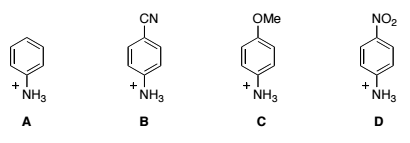# Problem: Put these compounds in order of  increasing acidity. a) A &lt; C &lt; B &lt; D b) C &lt; A &lt; B &lt; D c) B &lt; C &lt; D &lt; A d) D &lt; B &lt; A &lt; C e) D &lt; C &lt; A &lt; B

###### Problem Details

Put these compounds in order of  increasing acidity.

a) A < C < B < D

b) C < A < B < D

c) B < C < D < A

d) D < B < A < C

e) D < C < A < B